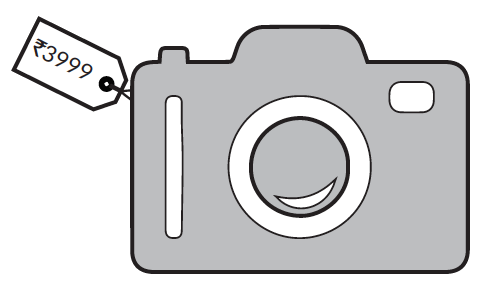# Taxes and Discounts

Go back to  'Commercial Math'

Taxes and discounts are a natural extension of the application of percentages. These are probably the most frequent terms that come up during a conversation about shopping since they directly affect the amount of money involved. To understand tax and discount better, it is important to know the related terms.

## Marked price

Almost every item comes with a price tag attached to it (usually, by the manufacturer of the item). This price is called the marked price, abbreviated as MP.

Let’s try and understand this better using an example.

The price tag on a camera reads $$₹3999$$ but it is being sold for $$₹3900.$$The marked price of the camera is $$₹3999.$$ The selling price is $$₹3900.$$

## Discount

We come across banners like “$$50 \% \text{ OFF}$$” or “$$75 \% \text{ OFF}$$” on a frequent basis. These are usually in different places to attract customers like us and are usually put up in malls or big stores. The difference between the selling price and marked price is called discount.

Example: Nikhil visited Levi's and bought a shirt for $$₹2450.$$ If the price tag on the shirt read $$₹2940.$$ What is  the amount of discount offered by the store on the shirt?

The marked price of the shirt is $$₹2940$$ and the selling price is $$₹2450.$$

\begin{align}\text{Discount}& = \text{Marked Price} - \text{Selling Price} \\ & = ₹2940 - ₹2450 \\ & = ₹490 \end{align}

We can calculate the percentage of discount on the marked price.

\begin{align}\text{Discount Percentage}& = {\text{Discount} \over \text{Marked Price}} \times 100 \\\\ & = {490 \over 2940} \times 100 \\\\ & = 16.66 \% \end{align}

## Tax

You must have heard words like income tax, GST, service tax etc in daily conversations. In recent years, GST was introduced, while other taxes like excise duty, VAT, municipal tax, entertainment tax etc. have existed for a while now. The government charges a fee on every item that is sold. This fee is meant to be spent on public welfare and is known as tax. The tax charged is always a percentage of the SP.

For example, let’s say an item cost $$₹500$$ and a sales tax of $$5 \%$$ was charged. What would be the bill amount?

Let’s try and find how much is $$5 \%$$ of $$500.$$

\begin{align}{5 \over 100} \times 500 = 25 \end{align}

Now let’s add this amount to the cost price.

\begin{align}₹500 + ₹25 = ₹525 \end{align}

The total bill amount including taxes would be $$₹525.$$

Let’s now try and understand how taxes are levied on a discounted product.

-  Discount is calculated on selling price excluding taxes.
-  Tax is applied on the amount arrived at after subtracting all the discount from selling price.

Example: Suppose you are ordering a Pizza at a Pizza parlour and it costs $$₹400.$$ The restaurant is offering a discount of $$30 \%$$ on all dine in orders, so the final amount that it will come to, assuming the restaurant only charges $$10 \%$$ GST as tax is:

Step 1 - Calculate the discounted price

\begin{align} \text{Discount} & = {30 \over 100} \times 400 \\ & = ₹120 \end{align}

\begin{align} \text{Final Bill Amount} & = ₹400 - ₹120 \\ & = ₹280 \end{align}

Remember the tax is levied on the discounted price. $$10 \%$$ GST was levied on the final amount

\begin{align} {10 \over 100} \times ₹280 = ₹28 \end{align}

\begin{align} \text{Final Amount} &= ₹280 + ₹28 \\ & = ₹308 \end{align}

## Tips and Tricks

• Be careful when applying successive discounts. Discount percentages cannot be simply added. E.g. Two successive discounts of $$5 \%$$ is not equal to one discount of $$10 \%.$$
For example, an item that costs $$₹2000$$ is discounted successively by $$5 \%.$$ First discount value will be \begin{align} 2000 \times (5 \div 100) = ₹100. \end{align} Now the price has already reduced to $$₹1900.$$ The second discount will be \begin{align} 1900 \times (5 \div 100) = ₹95. \end{align} So the final price of the item is $$₹1805.$$ As you can see, this turns out to be slightly more expensive than a single discount of $$10 \%.$$
• Remember that discounts are calculated on the marked price (or listed price) of an item. Taxes are calculated on the final discounted price of the item and not on the marked price.